# How to calculate derivative in Microsoft Excel

In this tutorial we will learn how to take derivatives using finite difference methods in Microsoft Excel. The finite difference method is a numerical method for solving differential equations by approximating them with a system of algebraic equations. It involves replacing the derivatives in a differential equation with finite differences, which are approximations of the true derivatives. These finite differences are calculated using a set of discrete data points and can be used to approximate the solution to the differential equation over a specified range. The finite difference method is commonly used in engineering and scientific applications for solving problems that cannot be solved analytically.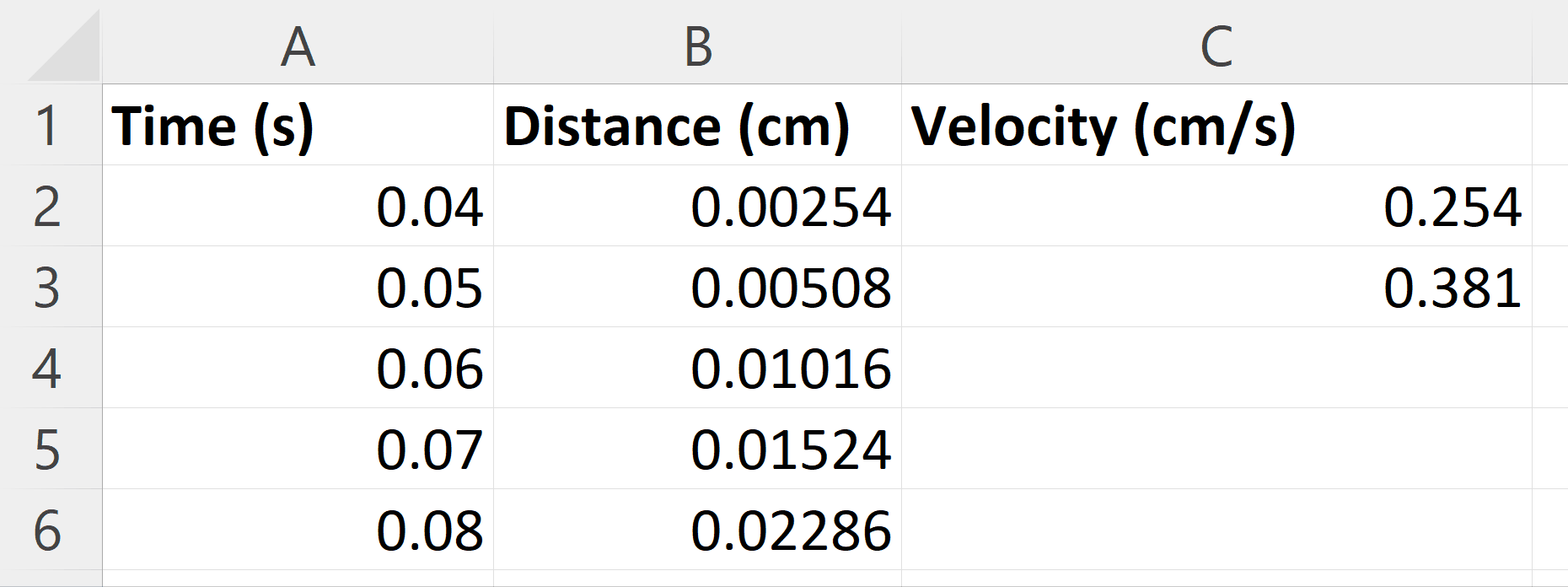Microsoft Excel is a spreadsheet software application that allows users to organize, format, and calculate data with formulas using a grid of cells arranged in rows and columns. It can be used for a variety of purposes such as creating budgets, tracking expenses, analyzing data, and much more. Excel provides a range of features including charts, pivot tables, and macros to make data analysis and visualization easier and more efficient.

### Step 1 – Arrange the Time and Distance data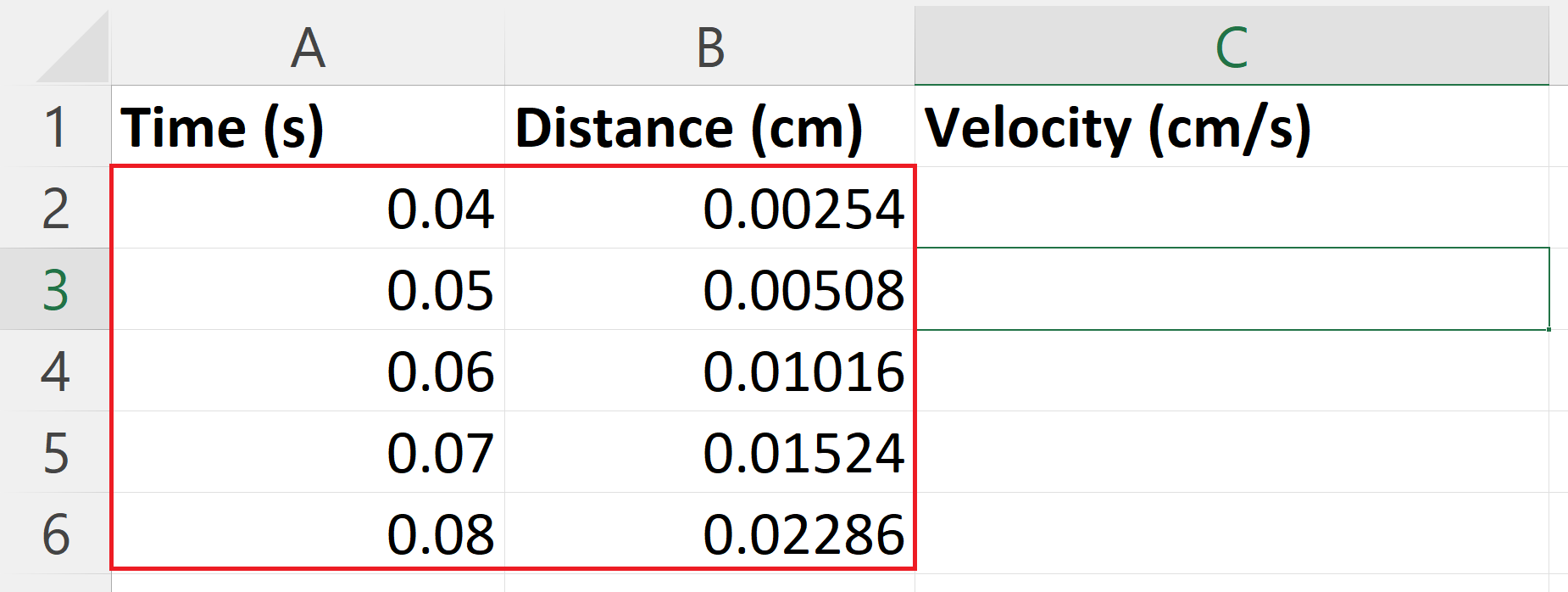– Arrange the Time and distance data in rows and columns so that it takes a tabular form.

### Step 2 – Select a Blank cell– Select a blank targeted cell where you want to take the derivative using a finite difference method.

### Step 3 – Place an Equals Sign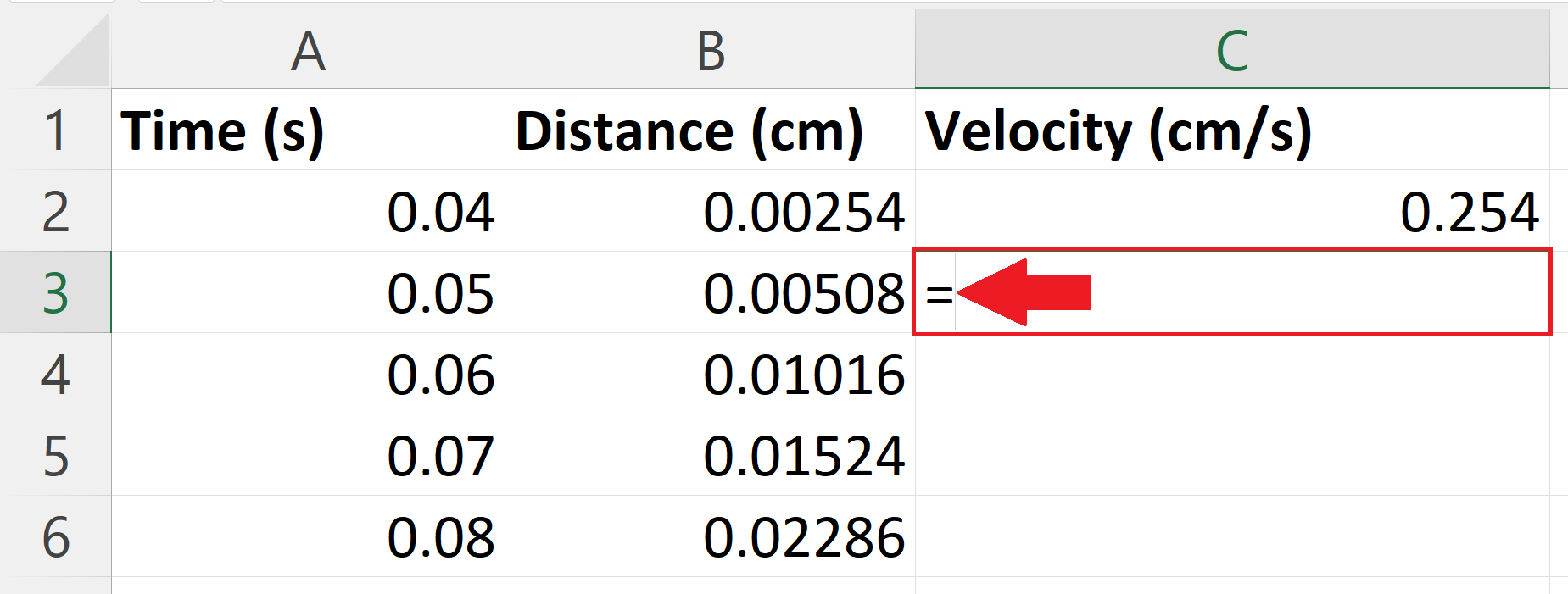– Place an Equals sign ( = ) in the blank targeted cell.

### Step 4 – Use the Finite Difference method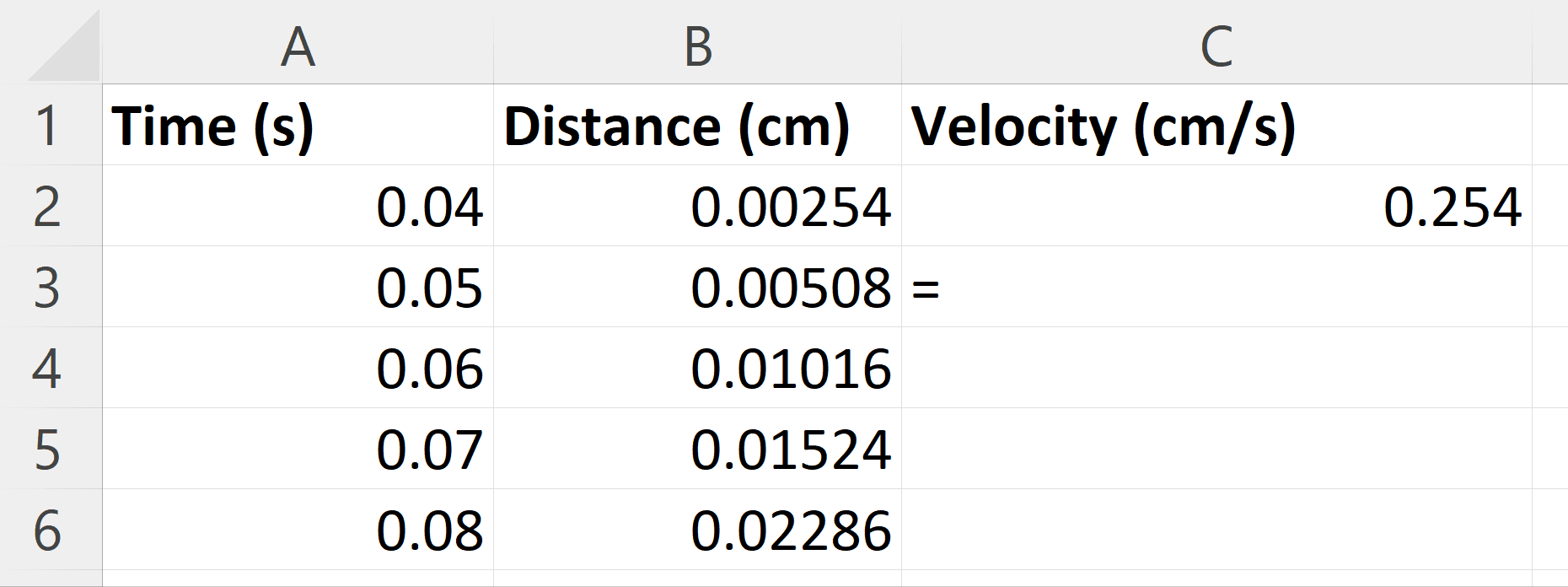– Here we will calculate the velocity by differentiating distance with respect to time using a finite difference method.
– The formula for finite difference method is
V(t)=(y2-y)/(x2-x)
– Where y2 and x2 are subsequent distance and time and y and x are preceding distance and time.

### Step 5 – Press the Enter Key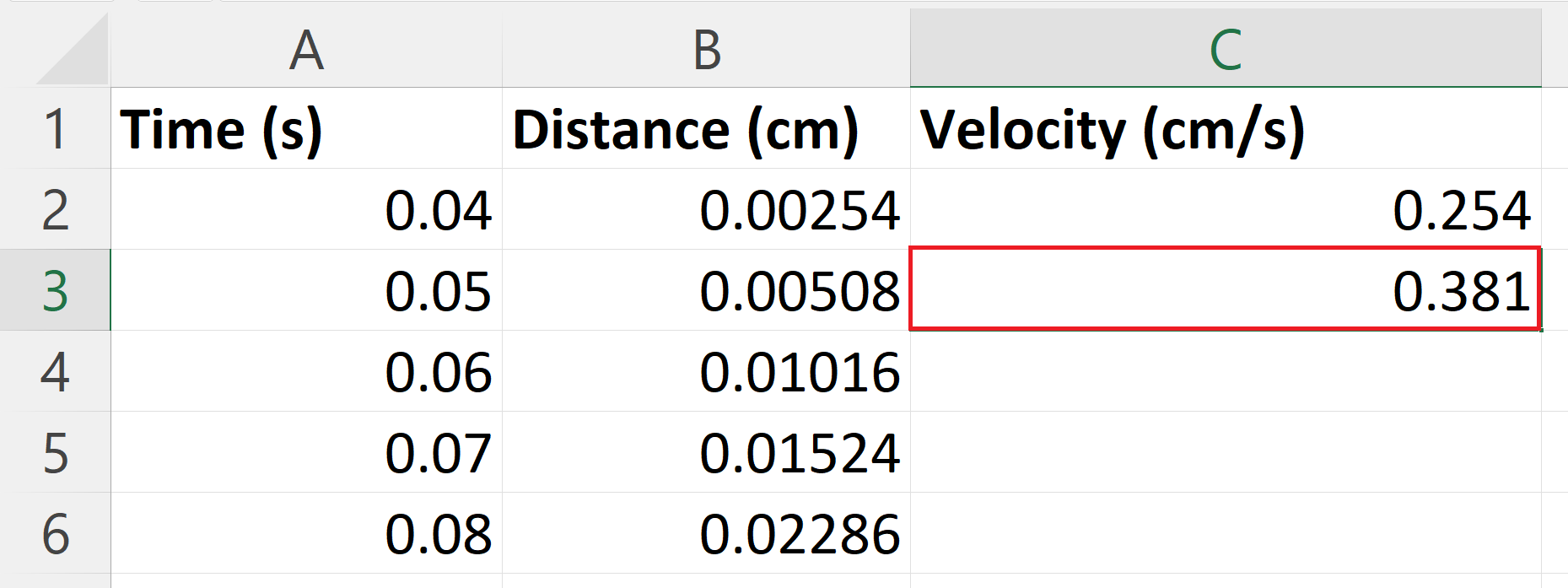– Press the enter key to get velocity i.e. derivative of distance with respect to time using finite difference method.

### Step 6 – Apply the Finite difference method on each Distance– Use the “Handle Select” and “Drag and Drop” method to apply the finite difference method on each distance.